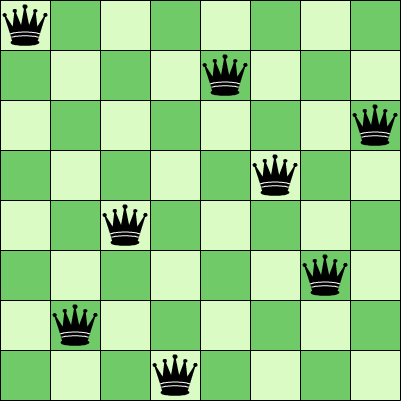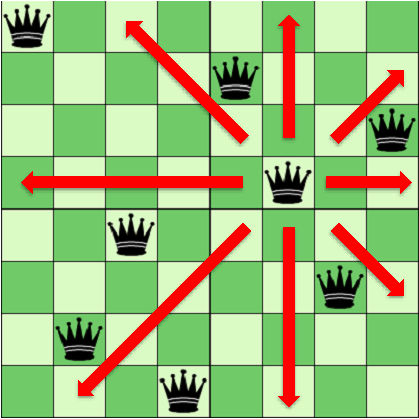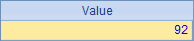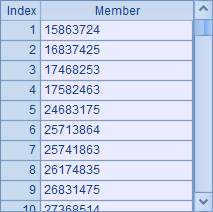# 趣味集算：八皇后问题

• 111
• 1 收藏，798 浏览A B C D 1 =*8 >i=1 2 for i>0 >A1(i)=A1(i)+1 3 if A1(i)==9 >A1(i)=0,i=i-1 next 4 if i==1 >i=2 next 5 =A1(i) =A1.to(i-1) 6 if C5.pos(B5)>0 next 7 else if C5.pselect(i-#==abs(B5-~))>0 next 8 >i=i+1 9 if i==9 >C1=C1 | A1.conj@s() >A1(8)=0,i=7 10 =C1.len()A B C D E 1 [] =func(A2,A1) =C1.len() 2 func for 8 if func(A5,A2,B2) =A2 | B2 3 if D2.len()==8 >C1=C1 | D2.conj@s() 4 else >func(A2,D2) 5 func if A5.pos(B5)>0 return false 6 for A5 if abs(B5-B6)==A5.len()+1-#B6 return false 7 return true

A5 中的子程序用来判断新的一行是否可以使用指定的列，其中在 C5 中查看是否本列已存在皇后，第 6 行查看是否已有皇后在同一斜行，在这样的情况下说明不能放置。如果某一列可以放置，则判断是否 8 个皇后都放置成功，如果已全部设置成功则记录在 C1 中。如果未放置满，则递归调用 A2 中的子程序，继续在下一行放置。这种方法的代码更易理解，计算结果与前面的方法是相同的。

• 111
• 1
• 798喜欢 | 1

• 0条回应给“趣味集算：八皇后问题”的评论